# How to Select All Error Value Cells in Excel

Sometimes we may get error values like #N/A or #NAME? in cells after applying formulas with errors. We can through pressing Ctrl key and clicking on each error value on worksheet to select all of them one by one. This way is time consuming and boring. So, if we want to select all of them at one time and clear them or replace them with comments, how can we do? This article will introduce you to select error value cells at the same time in a convenient way.

Precondition:

See screenshot below. We get some errors when applying VLOOKUP function to look up levels for people in table 1 refer to the source in table 2. We want to select all error cells now.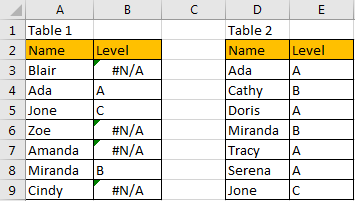## Method: Select All Error Value Cells Via Go To Special Function

Step 1: Select whole table 1 in worksheet.

Step 2: Click Home in the ribbon, then click the small triangle belongs to Find & Select icon in Editing group, then select Go To Special option.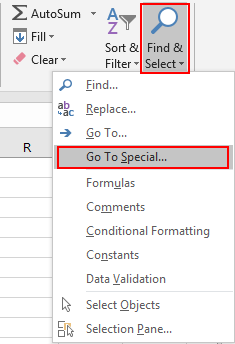Then Go To Special dialog displays.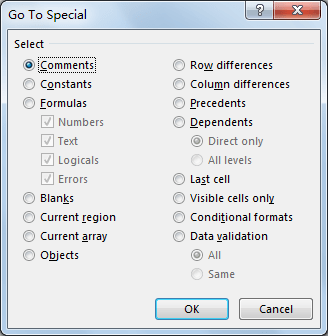In this step, you can also press hotkey F5 directly to trigger Go To dialog, then click on Special button on this dialog to load Go To Special dialog.Step 3: On Go To Special dialog, check on Formulas to enable Errors option, check on Errors, then click OK.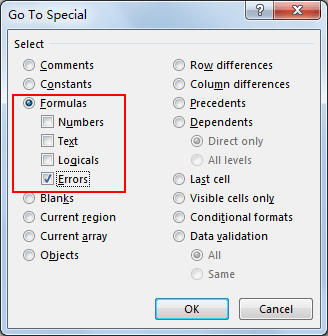Verify that all error value cells are selected.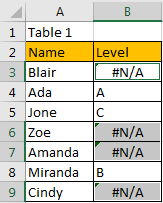If you want to clear errors or update comments, see below steps. If you just want to select all error value cells, you can ignore below steps.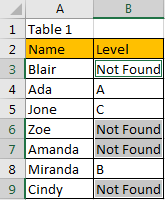Step 5: Clear error values. Directly press Backspace to delete error value in the first found error cell and then press Ctrl + Enter simultaneously. Then all errors are cleared.Related Posts

Calculate Number of Hours between Two Times

Calculating the difference between two times might be a valuable statistic for subsequent computations or averages, whether you're producing a time sheet for staff or recording personal exercises. While Excel has a plethora of complex functions, including date and time ...

Calculate Loan Interest in Given Year

When you borrow money, you are supposed to repay it gradually. Lenders, on the other hand, want to be compensated for their services and the risk they incur by lending you money. That is, you will not just repay the ...

Calculate Interest Rate for Loan

The interest rate is the fee charged by a lender to a borrower and is expressed as a percentage of the principal—the lent amount. The interest rate on a loan is often expressed as an annual percentage rate, abbreviated as ...

Calculate Interest for Given Period

Using the IPMT function in Excel, we can compute the interest payment on any loan. This step-by-step tutorial will guide Excel users of all skill levels through the process to calculate interest for given period. Finally, the formula: =IPMT(B3/12,1,B5,-B2) The ...

How To Use Excel GCD Function

This post will guide you how to use Excel GCD function with syntax and examples in Microsoft excel. Description The Excel GCD function Returns the greatest common divisor of two or more integers. So you can use the GCD function ...

Calculate A Ratio From Two Numbers In Excel

In elementary mathematics, a ratio is a connection or comparison between two or more integers. For example, ratios are often expressed as ":" to demonstrate the relationship between two numbers. You would think that manually calculating a ratio from two ...

How To Use Excel RRI Function

This post will guide you how to use Excel RRI function with syntax and examples in Microsoft excel. Description The Excel RRI function Returns an equivalent interest rate for the growth of an investment. So you can use the RRI ...

CAGR Formula Examples in Excel

CAGR in Excel is a formula that calculates the compound annual growth rate for any invested amount over the specified years or timeframe. Although there is no direct function in Excel that can help us identify the CAGR value, there ...

Build Hyperlink With VLOOKUP in Excel

You might have come across a task in which you were assigned to build hyperlinks, which seems very easy, and if you are new to excel or don't have enough experience with it, then you might wonder about doing this ...

Break ties with helper COUNTIF and column

Suppose you got a task to adjust the values that contain the ties; what would be your first attempt to break the ties of the given value? If you are wondering about doing this task manually, let me add that ...

Sidebar